# JEE(MAIN) Chemistry Mock Test - 1

## 30 Questions MCQ Test JEE Main Mock Test Series 2021 & Previous Year Papers | JEE(MAIN) Chemistry Mock Test - 1

Description
This mock test of JEE(MAIN) Chemistry Mock Test - 1 for JEE helps you for every JEE entrance exam. This contains 30 Multiple Choice Questions for JEE JEE(MAIN) Chemistry Mock Test - 1 (mcq) to study with solutions a complete question bank. The solved questions answers in this JEE(MAIN) Chemistry Mock Test - 1 quiz give you a good mix of easy questions and tough questions. JEE students definitely take this JEE(MAIN) Chemistry Mock Test - 1 exercise for a better result in the exam. You can find other JEE(MAIN) Chemistry Mock Test - 1 extra questions, long questions & short questions for JEE on EduRev as well by searching above.
QUESTION: 1

### Alcohols of low molecular weight are

Solution:

Alcohols are soluble in water. This is due to the hydroxyl group in the alcohol which is able to form hydrogen bonds with water molecules. Alcohols with a smaller hydrocarbon chain are very soluble. As the length of the hydrocarbon chain increases, the solubility in water decreases.

QUESTION: 2

### Benzyl alcohol is obtained from benzaldehyde by

Solution: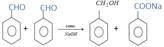QUESTION: 3

### The main structure features of proteins is

Solution:

The chemical bond formed between amino acids, constituting the primary linkagein all protein structures. In a peptide bond, the carboxyl group (COOH) of one amino acid bonds with the amino group (NH2) of another, forming the sequence CONH and releasing water (H2O).

QUESTION: 4

Chemical equilibrium is dynamic in nature, because

Solution:

A chemical equilibrium is dynamic in nature which in other words means that reactions continue to occur in both forward as well as backward direction with the same speed. As a result, the amount of product formed immediately reacts backward to give reactants and so there is no change in concentration of reactant or product with the passage of time.

QUESTION: 5

The indicator that is obtained by coupling the diazonium salt of sulphanilic acid with N,N-dimethylaniline is

Solution: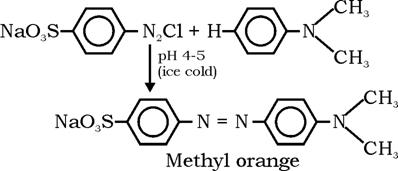QUESTION: 6

The standard e.m.f. of a galvanic cell, with standard electrode potentials of zinc equal to -0.76 V and that of copper equal to + 0.34 V, is

Solution:

Ecell = E right - E left
= E•Cu2+/Cu - E•Zn2+/Zn
= (+0.34v) - (-0.76v)
=0.34+0.76
=1.1v

QUESTION: 7
Which of the following is true for the order of a reaction?
Solution:
QUESTION: 8

When 10gram of methane is completely burnt in oxygen, the heat evolved is 560kJ. What is the heat of combustion (in kJ/mole) of methane?

Solution:

Heat of combustion of CH4 =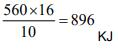QUESTION: 9

Which of the following is most electronegative?

Solution:

The electronegativity values for the representative elements decreses down a group because of the increase in atomic radius as well as screening effect. Thus, carbon has highest electronegativity in a group.

QUESTION: 10

The catalyst used for the polymerization of olefins is

Solution:

A Ziegler–Natta catalyst, named after Karl Ziegler and Giulio Natta, is a catalyst used in the synthesis of polymers of 1-alkenes (alpha-olefins).

QUESTION: 11

When one Faraday current is passed which of the following would deposit one gram atomic weight of the metal?

Solution:

Hint: For Na+, GEW=GAW

QUESTION: 12

A white salt is readily solubule in water and gives a colourless solution with a pH of about 9. The salt would be

Solution:

CH₃COONa undergoes anionic hydrolysis and gives basic solution (pH ≈ 9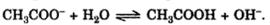QUESTION: 13

The pH of a solution of hydrochloric acid is 4. The molarity of this solution is

Solution:

The pH of a solution is equal to the base 10 logarithm of the H+ concentration, multiplied by -1. If you know the pH of a water solution, you can use this formula in reverse to find the antilogarithm and calculate the H+ concentration in that solution.

QUESTION: 14

Which of the following is least acidic?

Solution:
QUESTION: 15

Which one of the following can be considered as weak electrolyte ?

Solution:

CH3COOH does not ionise or dissociate completely and hence it is a weak electrolyte.

QUESTION: 16

Which of the following is not a Lewis acid ?

Solution:
QUESTION: 17

Which is not true about polymers?

Solution:

A polymer sample contains chains of varying lengths and therefore molecular mass is always expressed as an average.The molecular weight of polymers are very high,due to which they are expressed in two ways:-

Number average molecular mass and weight average molecular mass.

QUESTION: 18

A solid cube has CsCl type structure. If the edge length of the unit cell is 404 pm, what is the distance between A⁺ and B⁻ ions?

Solution:

In CsCl structure the distance the closest approach between the cation and anion is equal to half of the body of the diagonal of a cube.
Body diagonal = 3–√×3× Edge Length

=3–√×404

=699.74pm
The distance of the closest approach = 1/2× Body diagonal
=1/2×699.74 = 349.87 pm = 349.9pm

QUESTION: 19

Which of the following has maximum Schottky defect?

Solution:

More comparable the size of anion and cation, more is the chance of Schottky defect and Naand Cl- ions are almost comparable in size hence m ore probability to show  Schottky defect.

QUESTION: 20

Select the correct statement regarding the aromatic nitrogen molecule

Solution:

In pyrrole, the lone pair of electrons belonging to the nitrogen is part of the aromatic ring. However, in pyridine it is part of an sp2-hybridized orbital.

QUESTION: 21

On mixing a certain alkane with chlorine and irradiating it with ultraviolet light,it forms only one monochloroalkane .This alkane could be

Solution:

The neo-pentane has only one type of hydrogen atoms, i.e., primary hydrogen atoms. One Cl atom of Cl2 will substitute one H atom of these primary hydrogen atoms. Thus, only one type of mono-chloro substituted neo-pentane is formed.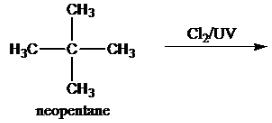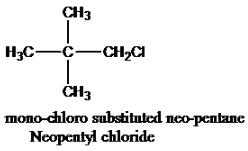QUESTION: 22

Acetic acid dissolved in benzene shows a molecular mass of

Solution:

Acetic acid (molar mass 60 g/mol) dimerises in benzene due to presence of hydrogen bonding between its molecule hence the molecular weight becomes double than that of normal value

QUESTION: 23

On dissolving 1 mole of each of the following acids in 1 litre water, the acid does not give a solution of strength 1 N is

Solution: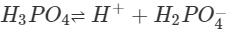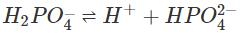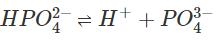Phosphoric acid does not give 1N strength.

QUESTION: 24

Catalyst used in dimerisation of acetylene to prepare chloroprene is

Solution: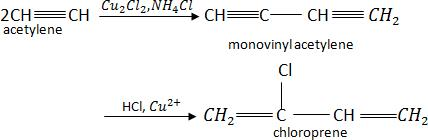QUESTION: 25

According to the Schrodinger's wave equation, the functuion (Ψn) of a particle in one dimensional box is ___

Solution:
QUESTION: 26

When one mole of the gas is heated at constant volume ,temperature get raised from 298 K to 308 K.Heat supplied to the gas is 500 J.Then which among the following is a correct statements ?

Solution:

As the gas is heated at constant volume. Therefore ΔV = 0, and W = 0 and at the same time q = ΔU

QUESTION: 27

Which element has a hydrogen like spectrum whose lines have wavelengths one fourth of atomic hydrgen?

Solution: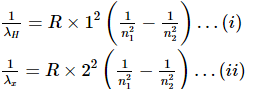Dividing equation (i) by equation (ii), we get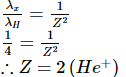QUESTION: 28

For several reactions an increase in temperature from 300K to 310K increases the rate approximately by__

Solution:

With rise in temperature by 10 K , Rate Constant nearly doubles, and hence Rate of the Reaction increases by 100%.

QUESTION: 29

Among the following ions which one has the highest paramagnetism?

Solution: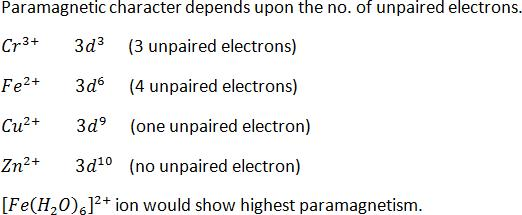QUESTION: 30

In Lassaigne's test for nitrogen, the blue colour is due to the formation of

Solution: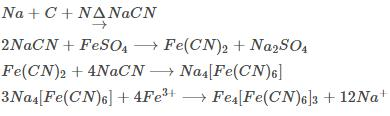It is ferric ferrocyanide.